Xprite Pixel Art EditorXprite is a pixel art editor designed for artists, game designers and programmers.

Xprite is under heavy development. Currently, a beta build is available for a discount. Don't miss out while it's still cheap :)Xprite

Goal

The mission of Xprite is to make drawing correct pixels less painful, so you can focus on creating art. We invented lots of algorithmic tools specifically for pixel art. If you think it's missing a feature, feel free to email us your requests.

Development

After 6 months of coding, Xprite is finally ready for release. There are still a few bugs left to fix and features to build. Place an order now to support development:

Preorder now

Wanted: Xprite Art

Finally, we are looking for a spectacular piece of pixel art created with Xprite to feature on the splash screen everytime Xprite boots up. Feel free to email us your best work.

Features

Easily color your contour to achieve 3d light effect.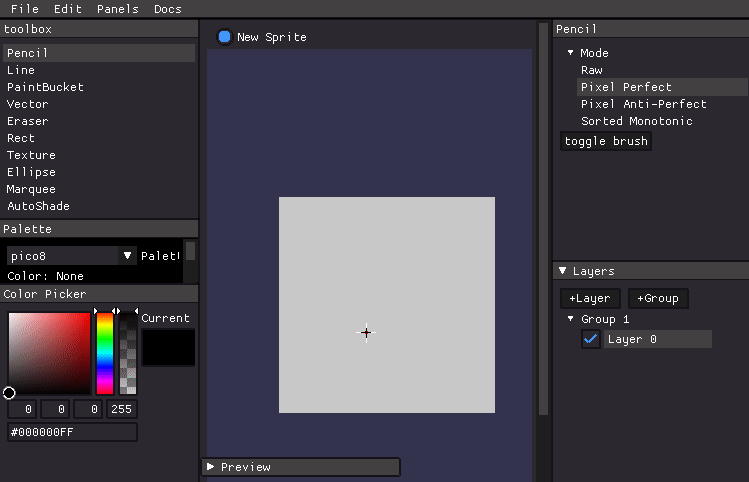Symmetry

Create symmetric patterns with fine-grained control.

Also, Xprite supports rotational symmetry.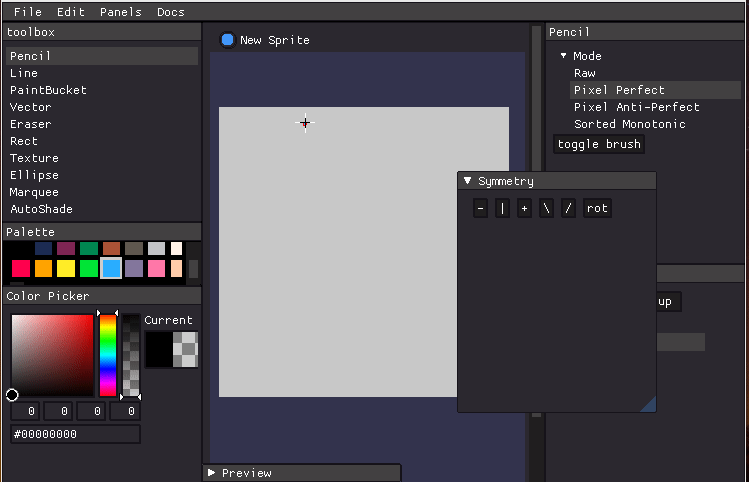Eight-way connected floodfill

This allows recoloring thin lines.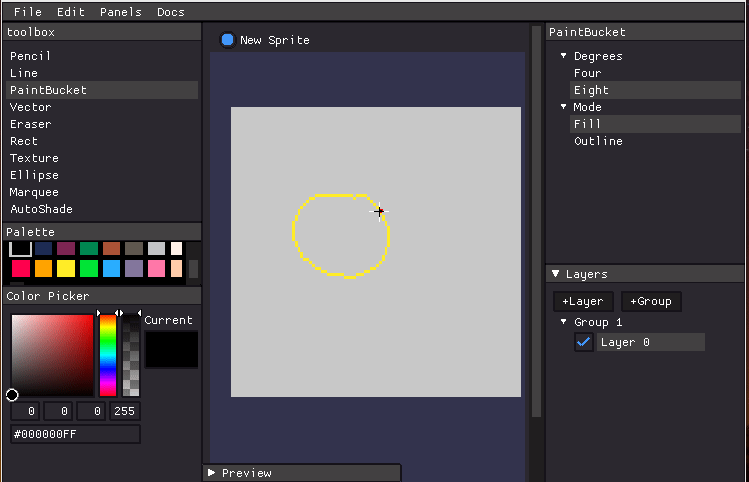Texture Generator

Uses wave function collapse algorithm to automatically generate intricate pixel patterns.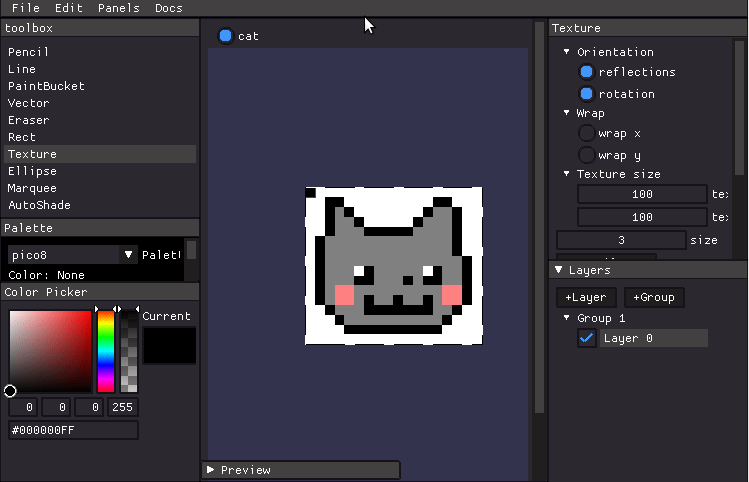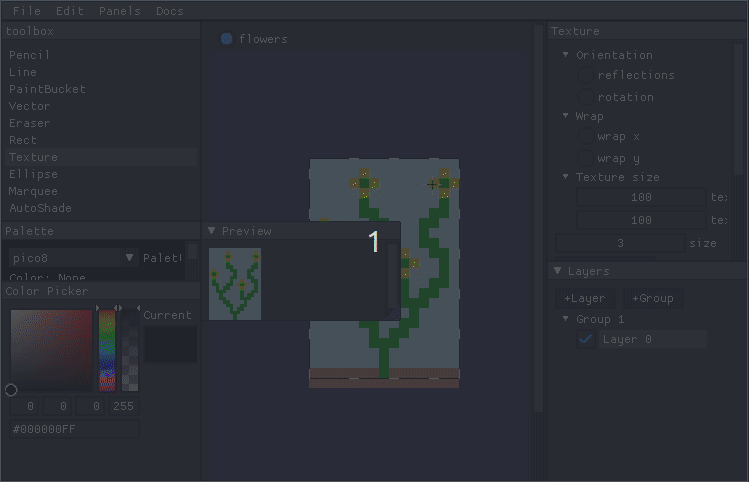Outline tool

Outline your color blobs to achieve a cartoonish effect.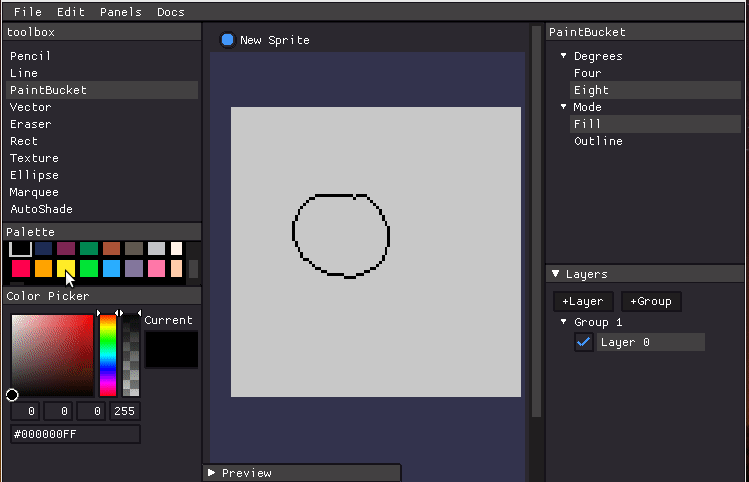Bezier Curves

Draw smooth curves by sorting monotonic curves by segment slope.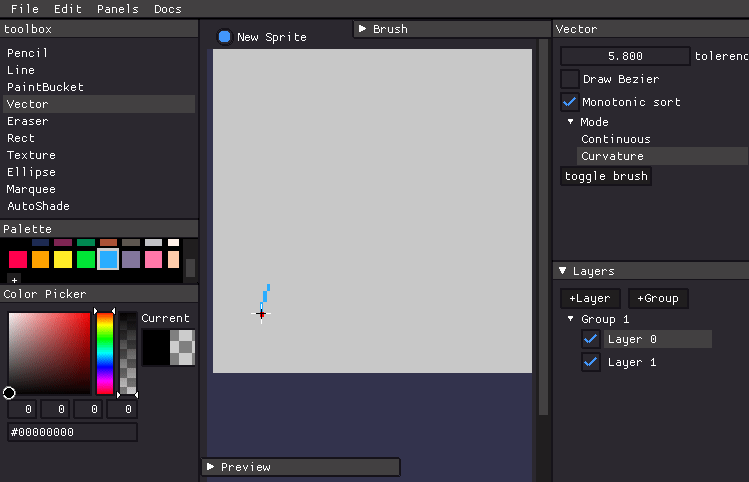Layer Exporter

Save each layer to a separate file.

Available formats: PNG, JPG, Twitter PNG, Aseprite, PSD.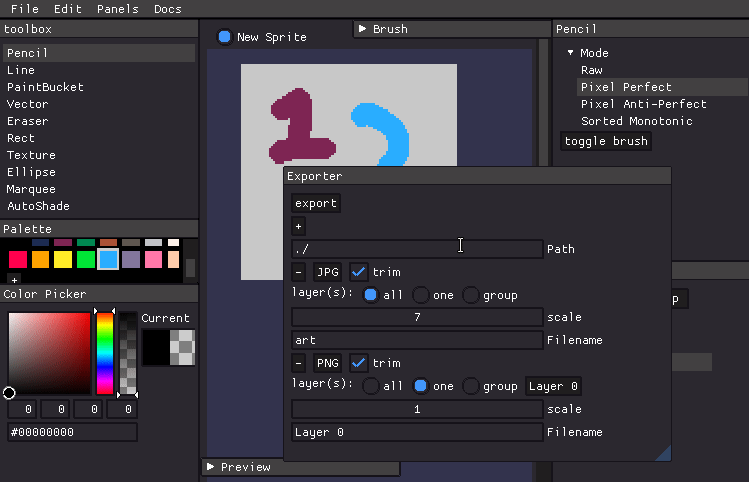pyckitup

Python game engine for the Web.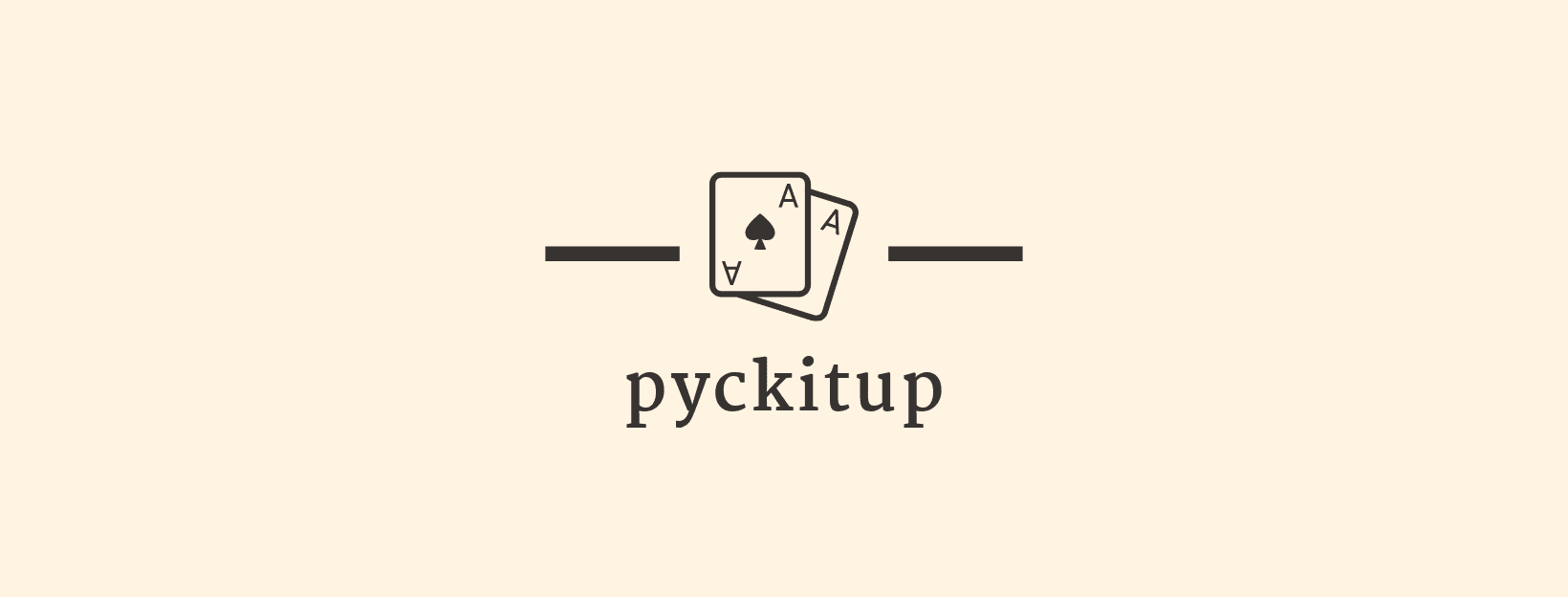Hi there! pyckitup is a Python game engine you can use to make 2D games. It is free, open source and works on Web, Linux, OS X and Windows.

Getting Started

1. Initialize game folder

The folder contains the clock example game.

``````pyckitup init hello
cd hello
pyckitup
``````

2. Once ready, deploy to web with

``````pyckitup build
``````

This creates a `build/` directory which contains everything you need to deploy your awesome game to the web. Simply copy the folder to where you want it served.

How it works

pyckitup is a thin layer glueing RustPython interpreter to quicksilver game engine. When you load a pyckitup game in browser, it loads a single 5MB wasm blob and interprets Python code stored in localStorage. As a result there is a 10MB code size limit.

Example: Clock

Source code:

``````import qs
from common import *

def init():
qs.init_sounds([ ["click", "click.wav"] ])
return {
"elapsed": 0,
"hours": 0,
"minutes": 0,
"seconds": 0,
}

qs.set_update_rate(1000)

def update(state):
state["elapsed"] += qs.update_rate()
elapsed = state["elapsed"]
state["seconds"] = (elapsed / 1000.) % 60.
state["minutes"] = ((elapsed / 1000.) / 60.) % 60.
state["hours"] = ((elapsed / 1000.) / 60. / 24.) % 24.

qs.sound("click")

def draw(state):
qs.clear(WHITE)

# draw the frame
qs.circ([400, 300], 203, color=BLACK)
qs.circ([400, 300], 200, color=WHITE)
# draw the hour markers
for i in range(1, 13):
angle = 360. * ((i + 9.) * 2. / 24.)
rad = angle * 3.14 / 180
pos = [ math.sin(rad) * 200. + 400., math.cos(rad) * 200. + 300. ]
qs.line([[400, 300], pos], thickness=5, color=BLACK)
qs.circ([400, 300], 180, color=WHITE)

hour_angle = 360. * ((state["hours"] + 9.) * 2. / 24.) * 3.14 / 180
minute_angle = 360. * ((state["minutes"] + 45.) / 60.) * 3.14 / 180
second_angle = 360. * ((state["seconds"] + 45.) / 60.) * 3.14 / 180

hour_pos =   [math.cos(hour_angle  ) * 150. + 400, math.sin(hour_angle)* 150 + 300]
min_pos =    [math.cos(minute_angle) * 180. + 400, math.sin(minute_angle) * 180+ 300]
second_pos = [math.cos(second_angle) * 180. + 400, math.sin(second_angle) * 180. + 300]

qs.line([[400, 300], hour_pos], thickness=10, color=BLACK)
qs.line([[400, 300], min_pos], thickness=5, color=BLUE)
qs.line([[400, 300], second_pos], thickness=3, color=RED)
``````

Example: Color

Source code:

``````import qs
from common import *

def init():
qs.init_anims([
# name, path, nframes, duration(s)
["crab-up", "crab-up.png", 2, 1.],
])
return {
"p0": [1., 1.],
"p1": [100., 100.],
"color": [0,0,0,1],
}

def update(state):
state["p0"] += 0.3
state["p0"] += 0.3

state["color"] += 0.001
state["color"] += 0.002
state["color"] += 0.003
state["color"] %= 1
state["color"] %= 1
state["color"] %= 1

def draw(state):
qs.clear(state["color"])
qs.anim("crab-up", rect=[state["p0"], state["p1"]])

def event(state, event):
if event["event"] == "mouse_moved":
state["p0"] = event["x"]
state["p0"] = event["y"]
``````

API

Lifecycle functions

The lifecycle of a pyckitup game consists of these functions:

1. `def init() -> State`

Initializes assets, returns a state object which can be an integer, a list, a dictionary or practically any Python object.

1. `def onload(State) -> None`

This function is run exactly once after window creation. Can be used for setting frame rate, getting window size, etc..

1. `def update(State) -> None`

This function is guaranteeed to run once per frame.

1. `def draw(State) -> None`

Draw your game visuals here. In contrast to update function above, there is no once-per-frame guarantee.

1. `def event(State, Event) -> None`

Event is a dictionary containing the event type and its associated data.

Assets

All assets must be placed in the `static/` folder. Assets may include sprites, sprite animations and sounds. Assets must be declared in the init lifecycle function before use. asset loading is asynchronous and pyckitup will panic if not all assets are loaded.

The asset initializer functions are detailed in the qs module section.

Directory Structure

``````/game
|-run.py
|-module.py
|-/static        <- place your assets here
| |-sprite.png
| |-anim.png
| |-sound.wav
``````

Events

Event objects are dispatched in the `event` lifecycle function:

``````def event(state, event):
print(event)
``````
• Closed
``````{
"event": "closed"
}
``````
• Focused
``````{
"event": "focused"
}
``````
• Unfocused
``````{
"event": "unfocused"
}
``````
• Key
``````{
"event": "key",
"key": "A",
"state": "Pressed"
}
``````
• Typed
``````{
"event": "typed",
"char": "A"
}
``````
• MouseMoved
``````{
"event": "mouse_moved",
"x": 0,
"y": 0
}
``````
• MouseEntered
``````{
"event": "mouse_entered"
}
``````
• MouseExited
``````{
"event": "mouse_exited"
}
``````
• MouseWheel
``````{
"event": "mouse_wheel",
"x": 0,
"y": 0
}
``````
• MouseButton
``````{
"event": "mouse_button",
"button": "Left",
"state": "Pressed"
}
``````

Buttons

``````Key1
Key2
Key3
Key4
Key5
Key6
Key7
Key8
Key9
Key0
A
B
C
D
E
F
G
H
I
J
K
L
M
N
O
P
Q
R
S
T
U
V
W
X
Y
Z
Escape
F1
F2
F3
F4
F5
F6
F7
F8
F9
F10
F11
F12
F13
F14
F15
F16
F17
F18
F19
F20
F21
F22
F23
F24
Snapshot
Scroll
Pause
Insert
Home
Delete
End
PageDown
PageUp
Left
Up
Right
Down
Back
Return
Space
Compose
Caret
Numlock
AbntC1
AbntC2
Apostrophe
Apps
At
Ax
Backslash
Calculator
Capital
Colon
Comma
Convert
Decimal
Divide
Equals
Grave
Kana
Kanji
LAlt
LBracket
LControl
LShift
LWin
Mail
MediaSelect
MediaStop
Minus
Multiply
Mute
MyComputer
NavigateForward
NavigateBackward
NextTrack
NoConvert
OEM102
Period
PlayPause
Power
PrevTrack
RAlt
RBracket
RControl
RShift
RWin
Semicolon
Slash
Sleep
Stop
Subtract
Sysrq
Tab
Underline
Unlabeled
VolumeDown
VolumeUp
Wake
WebBack
WebFavorites
WebForward
WebHome
WebRefresh
WebSearch
WebStop
Yen
``````

ButtonState

• Pressed

The button was activated this frame

• Held

The button is active but was not activated this frame

• Released

The button was released this frame

• NotPressed

The button is not active but was not released this frame

`qs` module

`qs` module is the namespace for quicksilver library functions:

• draw(shape, sprite and sprite animation)

• play sound

• initialize asset

• get mouse position, keyboard state, etc..

The name `qs` comes from the underlying game framework `quicksilver`.

pyckitup Types

The types in pyckitup are simple Python objects.

• Point: A cartesian point represented as a list `[x, y]`.

• Color: Linear RGBA type. It is a list of four floats with range [0., 1.]. Example: `BLUE = [0, 0, 1, 1]`

• Transform: 3x3 Transformation matrix. a list of lists that represents a 3x3 matrix with the last being homogeneous coordinate.

``````def rotate(deg):
theta = deg * 3.14 / 180.
c = math.cos(theta)
s = math.sin(theta)
return [
[c,-s, 0],
[s, c, 0],
[0, 0, 1]
]

def scale(x, y):
return [
[x, 0, 0],
[0, y, 0],
[0, 0, 1]
]

def matmul(X, Y):
return [[sum(a*b for a,b in zip(X_row,Y_col)) for Y_col in zip(*Y)] for X_row in X]
``````

Draw

All draw calls have these common optional named arguments:

``````qs.rect(.., color: Color, transform: Transform, z: int)
``````

These are the default values for the optional arguments:

``````Color: Red
Transform: Identity
z: 0
``````

Shapes

Rectangle

``````qs.rect([Point, [w, h]], ..)
``````

Example:

``````# Draw a blue rectangle with a top-left corner at
# (100, 100) and a width and height of 32
qs.rect([[100,100], [32,32]], color=BLUE)
``````

Circle

``````qs.circ([Point], radius: float, ..) # radius is required
``````

Example:

``````# Draw a green circle with its center at (400, 300) and a radius of 100
qs.circ( [400, 300], 100., color=GREEN)
``````

Triangle

``````qs.triangle([Point, Point, Point], ..)
``````

Example:

``````# Draw a red triangle rotated by 45 degrees, and scaled down to half
qs.triangle([[500, 50], [450, 100], [650, 150]],
color=RED, transform=matmul(rotate(45), scale(0.5, 0.5)), z=0)
``````

Line

``````qs.line([Point, Point], thickness: int, ..) # thickness is an optional named argument with default=1
``````

Example:

``````# Draw a red line with thickness of 2 pixels and z-height of 5
qs.line([[50, 80], [600, 450]], thickness=2., color=RED, z=5)
``````

Assets

Sprites are uniquely identified by name and must be initialized in the `init` lifecycle function.

Image drawing functions can be called in two ways:

1. `.., rect=[Point, Point], ..`

Use this to draw your sprite in the specified bounding rectangle.

1. `.., p0=Point, ..`

Align the top left corner of the sprite with p0 and leave width and height unchanged.

Sprite

``````qs.sprite(sprite_name: str, rect=[Point, Point], ..)
# or
qs.sprite(sprite_name: str, p0=Point, ..)
``````

Animation

``````qs.anim(animation_name: str, rect=[Point, Point], ..)
# or
qs.anim(animation_name: str, p0=Point, ..)
``````

Sound

Sound is also unique identified by name. This function plays the sound:

``````qs.sound(name: str)
``````

Initializers

Sprites

``````args: [(name, path)]
``````

Sprites Animations

``````args: [(name, path, number of frames, duration(in s))]
``````

Sounds

``````args: [(name, path)]
``````

Example:

``````qs.init_sprites([
["crab", "crab.png"], # name, path
])
qs.init_anims([
["crab-left", "crab-left.png", 2, 1.], # number of frames, duration(in s)
])
qs.init_sounds([
["click", "click.wav"]
])
``````

misc

``````qs.mouse_pos()
qs.keyboard()
qs.keyboard_bool()
qs.mouse_wheel_delta()
qs.set_view()
qs.set_update_rate()
qs.update_rate()
qs.clear()
``````

Contributing

pyckitup mainly uses RustPython and quicksilver to do all the heavy lifting. Most of pyckitup is glue between these two crates.

Quicksilver supports both native and web backends so the idea is to include a python interpreter and glue the game loop to Python functions.

``````cargo install cargo-web --git https://github.com/rickyhan/cargo-web --force
# git clone https://github.com/rickyhan/RustPython
git clone git@github.com:pickitup247/pyckitup.git
cd pyckitup
cargo web deploy --release
cargo build --release
``````

TODO

[ ] Implement font rendering.

Contact

Email: pickitup247@rickyhan.com

GitHub issues

GitHub issues